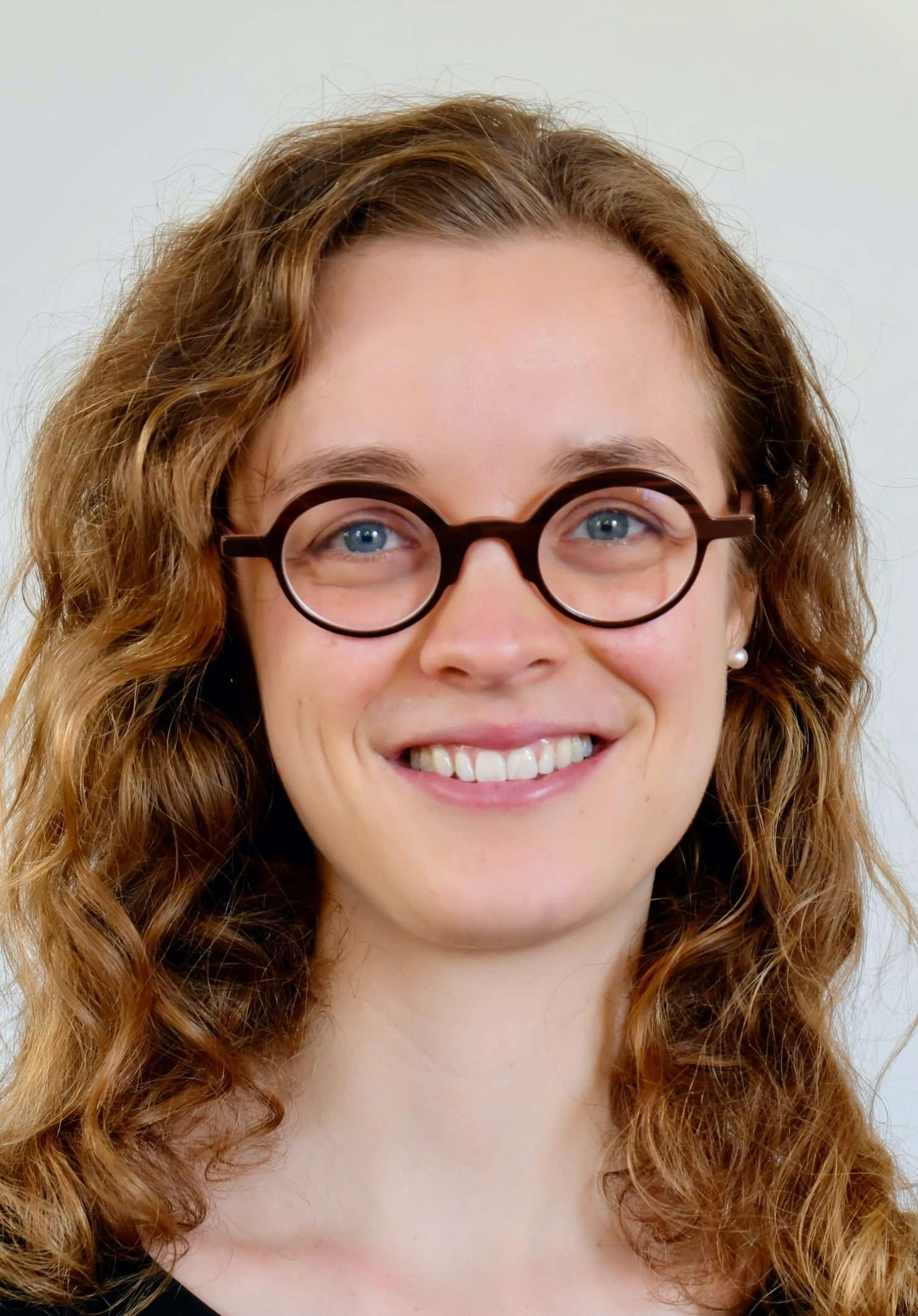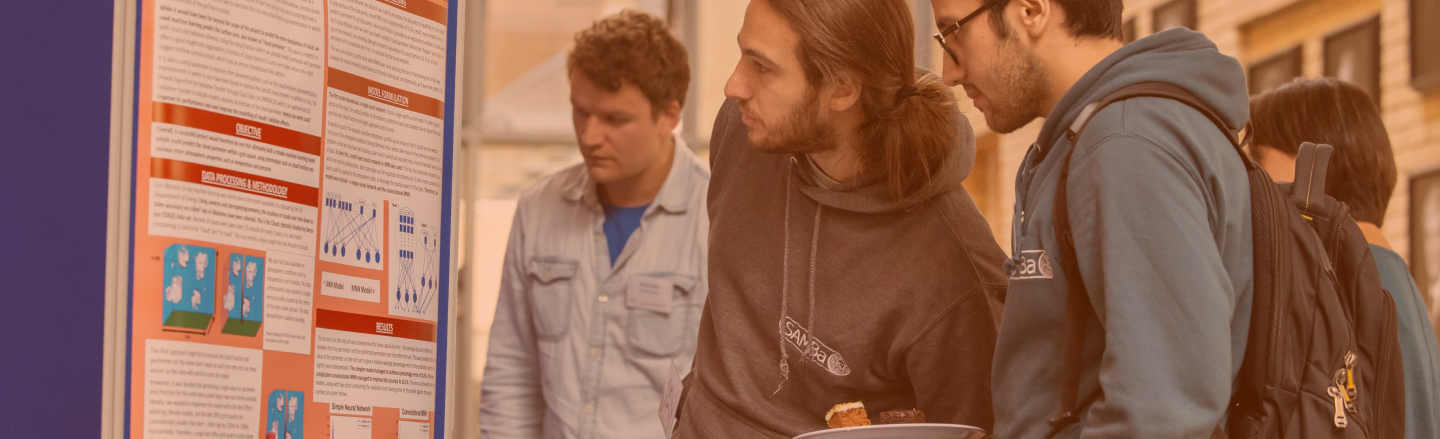#### Lisa Kreusser

###### Lecturer (Assistant Professor), University of Bath

I am a Lecturer (Assistant Professor) at the Department of Mathematical Sciences and a fellow at the Institute for Mathematical Innovation at the University of Bath.

My research is at the interface of partial differential equations, data analysis and mathematical formulations for machine learning. I am interested in combining modelling, analysis, partial differential equations, applied dynamical systems and numerical analysis to nonlinear problems with applications in biology, climate modelling, engineering and industry.